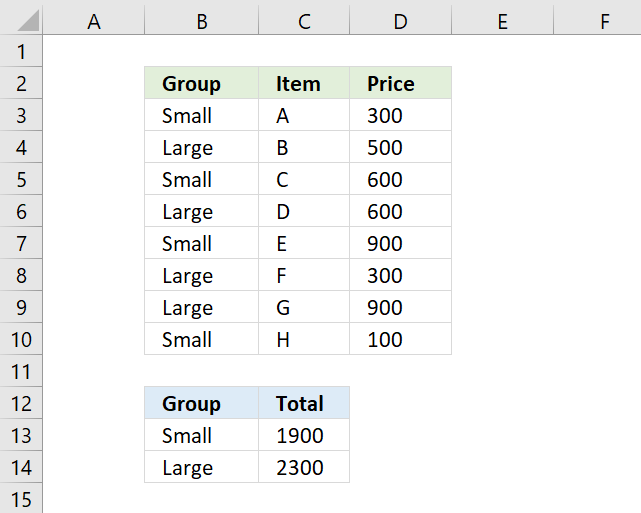Author: Oscar Cronquist Article last updated on March 25, 2018To extract groups from cell range B3:B10 I use the following regular formula in cell B13.

=LOOKUP(2,1/(COUNTIF(\$B\$12:B12,\$B\$3:\$B\$10)=0),\$B\$3:\$B\$10)

Copy cell B13 and paste to cell B14. Read this article to learn how this formula works.

To sum the numbers by group or value simply use this formula in cell C13.

=SUMIF(\$B\$3:\$B\$10,B13,\$D\$3:\$D\$10)

Copy cell C13 and paste to cell C14.

### Explaining formula

The SUMIF function sums cells that meet a condition, the first argument is the range you want to apply the criteria on. In this case cell range \$B\$3:\$B\$10, the \$ (dollar signs) make the cell reference locked so when the formula is copied the reference stays the same.

The second argument is the criteria found in cell C13. That cell reference has no \$ (dollar sign) so it changes when the formula is copied to the cell below. Why? We want it to use another group as a criterion in the next cell.

The third argument \$D\$3:\$D\$10 is the actual values we want to sum since they point to the same cell reference no matter where the formula is. It has a \$ (dollar sign) that make it an absolute cell reference.

I highly recommend a pivot table if you have lots of data to work with.

### Get excel *.xlsx file

Sum by group.xlsx